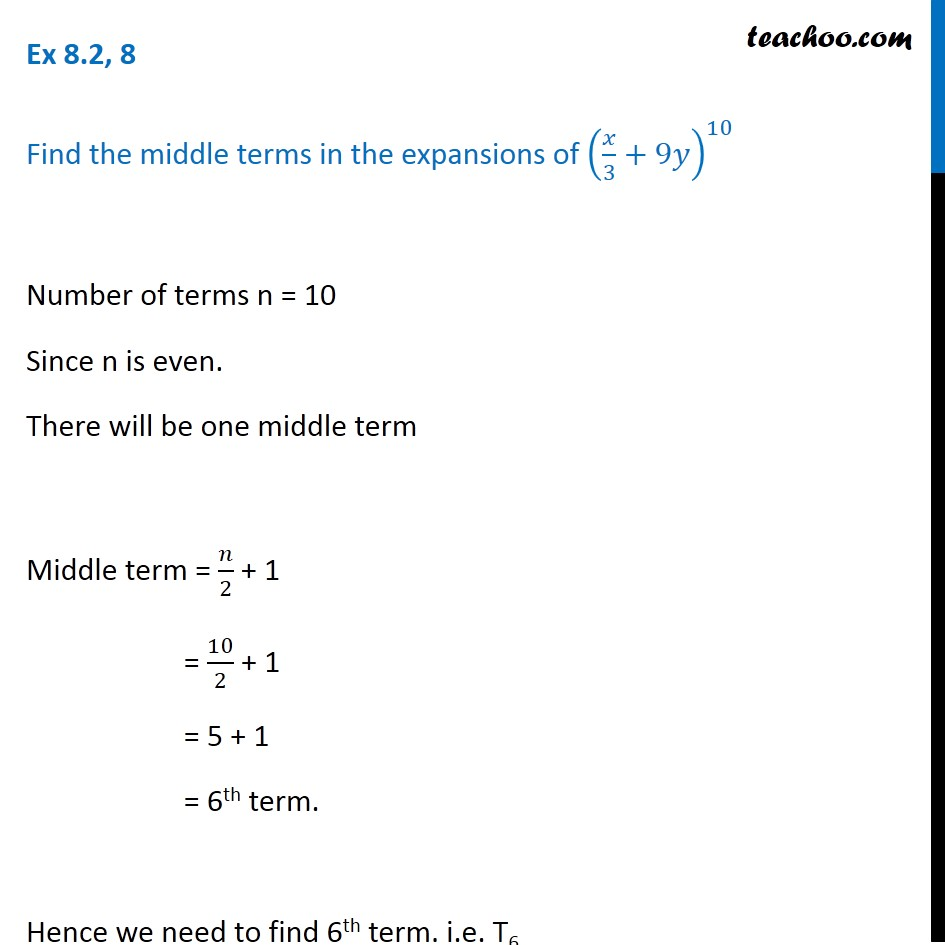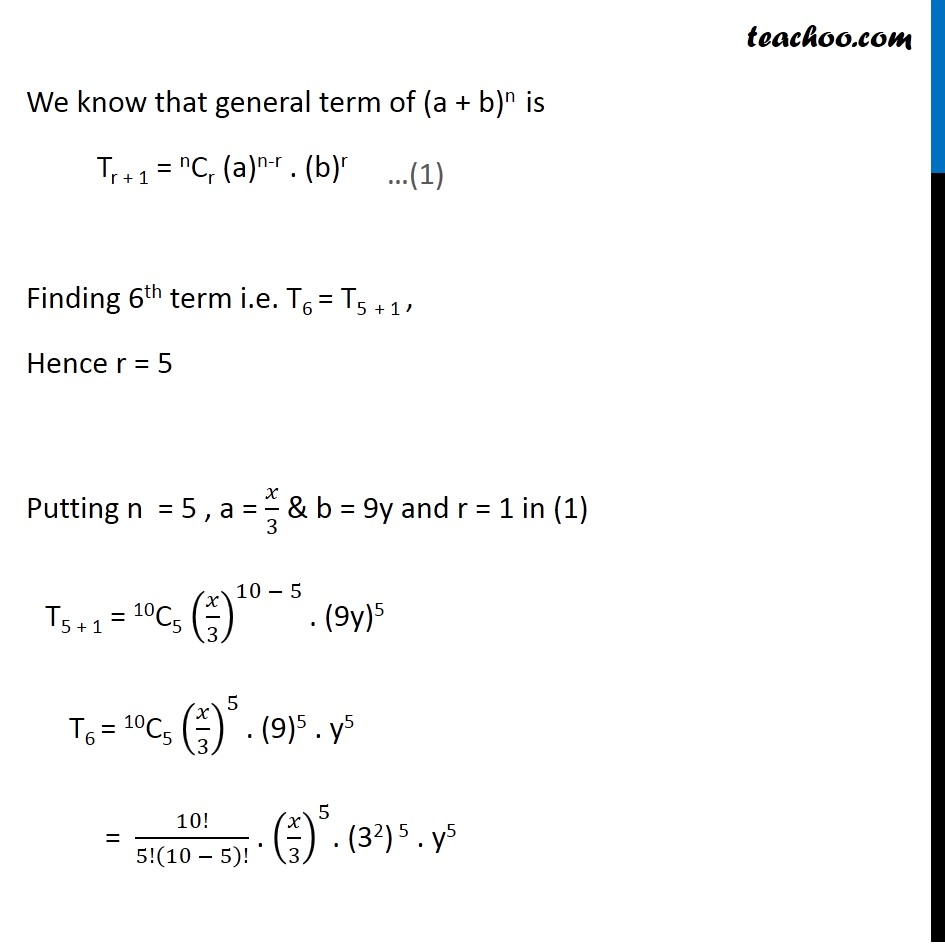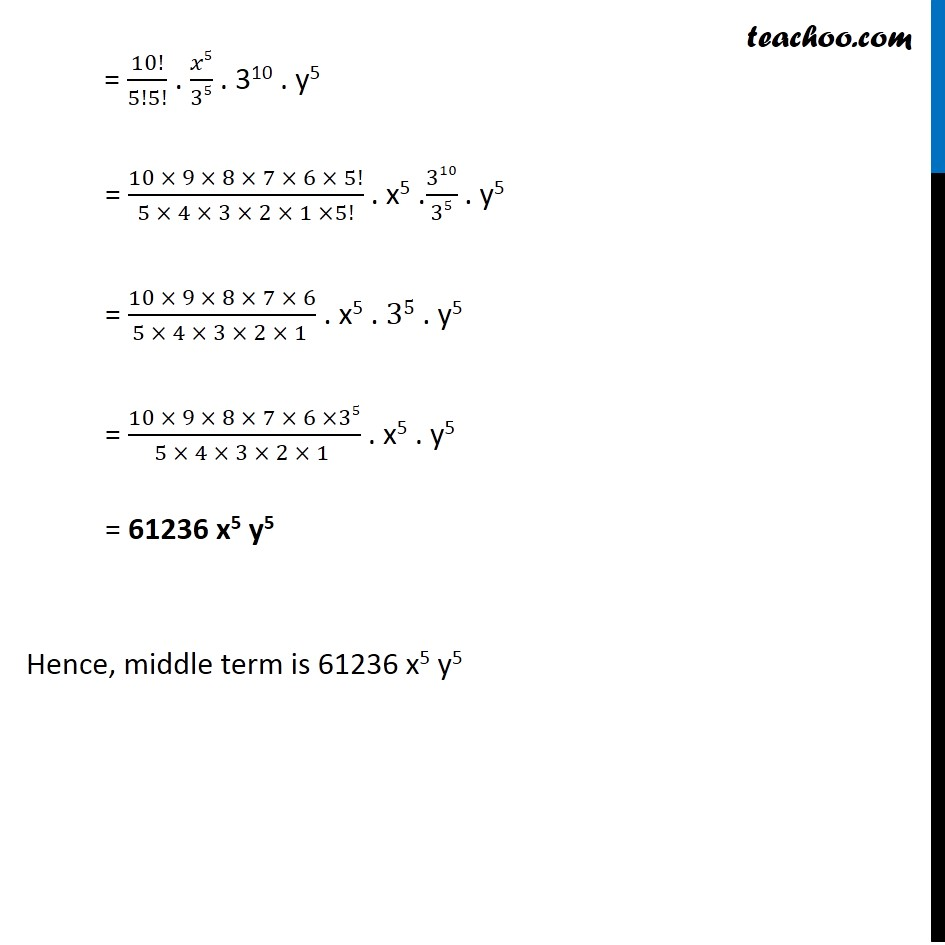General Term of Binomial Theorem

Chapter 7 Class 11 Binomial Theorem
Serial order wiseLearn in your speed, with individual attention - Teachoo Maths 1-on-1 Class

### Transcript

Question 8 Find the middle terms in the expansions of (𝑥/3+9𝑦)^10 Number of terms n = 10 Since n is even. There will be one middle term Middle term = 𝑛/2 + 1 = 10/2 + 1 = 5 + 1 = 6th term. Hence we need to find 6th term. i.e. T6 We know that general term of (a + b)n is Tr + 1 = nCr (a)n-r . (b)r Finding 6th term i.e. T6 = T5 + 1 , Hence r = 5 Putting n = 5 , a = 𝑥/3 & b = 9y and r = 1 in (1) T5 + 1 = 10C5 (𝑥/3)^(10 − 5) . (9y)5 T6 = 10C5 (𝑥/3)^5 . (9)5 . y5 = 10!/5!(10 − 5)! . (𝑥/3)^5. (32) 5 . y5 = 10!/5!5! . 𝑥5/35 . 310 . y5 = (10 × 9 × 8 × 7 × 6 × 5!)/(5 × 4 × 3 × 2 × 1 ×5!) . x5 .310/35 . y5 = (10 × 9 × 8 × 7 × 6)/(5 × 4 × 3 × 2 × 1 ) . x5 . 35 . y5 = (10 × 9 × 8 × 7 × 6 ×35)/(5 × 4 × 3 × 2 × 1 ) . x5 . y5 = 61236 x5 y5 Hence, middle term is 61236 x5 y5